## Tuesday, August 1, 2017

### Changing Demographics

In the United States, the mathematically intensive disciplines—engineering, the mathematical sciences, and the physical sciences—have traditionally been dominated by White males. It is common knowledge that the U.S. population is changing. Data from the National Center for Education Statistics (NCES) of the Department of Education  show that while 73% of high school graduates in 1995 were White, by 2015 that had decreased to 55%, on track to drop below 50% by 2025, in just eight years (Figure 0.1). These and all data in this article are taken from the NCES Digests of Education Statistics, 1990 through 2017, available at nces.ed.gov/programs/digest/

It has become a truism that if the United States is to maintain its pre-eminence in science and technology, we must ensure that traditionally underrepresented minorities share in this preparation for mathematically intensive careers. Groups like the Mathematical Association of America have programs such as the Tensor grants that encourage students from underrepresented groups to succeed in mathematics.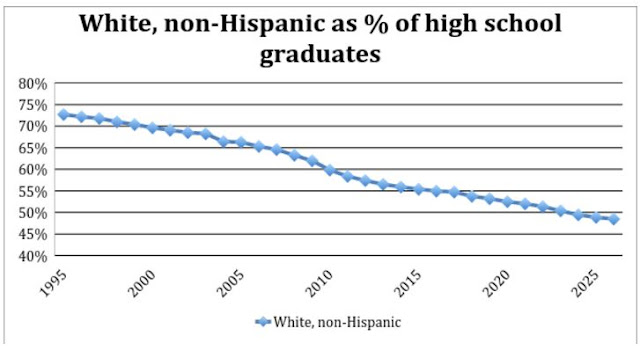Figure 0.1. White non-Hispanic students as percentage of all high school graduates. Percentages after 2012 are estimates based on the number of students already in the K-12 pipeline. Note: scale starts at 40%.

While we have seen and should continue to see a modest increase in the percentage of Asian and Black students, most of the changing demographics are shaped by the dramatic growth in the number of Hispanic students, which grew from 9% of high school graduates in 1995 to 21% in 2015, projected to reach 27% by 2025 (Figure 0.2).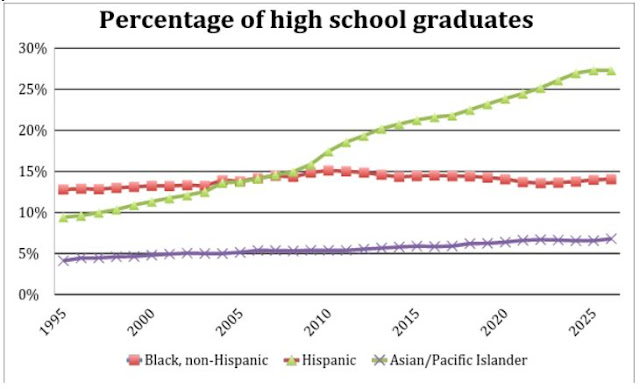Figure 0.02  Black non-Hispanic, Hispanic, and Asian/Pacific Islander students as a percentage of all high school graduates. Percentages after 2012 are estimates based on the number of students already in the K-12.

The intent of this article is simply to exhibit the data showing how well we are including various racial, ethnic, and gender groups among the recipients of bachelor’s degrees in engineering, the mathematical sciences, and the physical sciences. In future articles, I will address some of the ways in which MAA and other organizations are addressing the issues raised by these trends.

The bulk of this paper is taken with three appendices that show the graphs of the percentage of bachelor’s degrees earned in these three areas by each of the following demographic groups:

• Figure x.1. Women
• Figure x.2. White students, also reported by gender
• Figure x.3. Black students, also reported by gender Figure x.4.
• Hispanic students, also reported by gender
• Figure x.5. Asian students, also reported by gender
• Figure x.6. Non-resident alien students, also reported by gender where x is 1 for engineering, 2 for the mathematical sciences, and 3 for the physical sciences.
Native Americans/Alaskan Natives account for 1.1% of high school graduates, 0.3% to 0.5% in engineering and mathematics, and 0.5% to 0.8% in the physical sciences. These numbers are so small that there is tremendous year-to-year variation, and the graphs do not exhibit meaningful trends. Only in 2011 did NCES begin separating Asian from Pacific Islander and begin to allow students to self-identify as two or more races. For the sake of consistency, all of the data reported for Asian students include Pacific Islander students. In the disciplines of engineering, mathematical sciences, and physical sciences, Pacific Islanders make up between 0.1% and 0.2% of the total majors. In the first year that the choice of two or more races was allowed, about 1% of the students in each of the three disciplines so identified. This had risen to 3% by 2015. From 2011 through 2015, about 2% of high school graduates identified as two or more races.

Observations

Probably the most striking graph in this entire collection is Figure 2.6, showing the proportion of mathematics degrees going to non-resident aliens. Historically, this has been around 4%. It began to take off in 2008. By 2015, 13% of the bachelor’s degrees in the mathematical sciences were awarded to non-resident aliens. While we welcome these visitors and hope that many of them will stay, it is disturbing that so much of our mathematical talent must be imported. As shown in Figures 1.6 and 3.6, there have also been increases in the fraction of engineering and physical science degrees earned by non-resident aliens, but here the growth has not been nearly as dramatic.

A very disturbing set of graphs are given in Figures 1.3, 2.3, and 3.3, showing the proportion of degrees earned by Black students. In all three disciplines, we see a pattern of substantial growth during the 1990s, followed by a period of leveling off, followed by substantial decline. In engineering and the physical sciences, the percentage of degrees earned by Black students has dropped to levels not seen since 1993. In mathematics, the percentage of degrees awarded to Black students in 2015, 4.6%, is below that of 1990, when it was 5.0%.

The graphs showing the percentage of women in these fields, Figures 1.1, 2.1, and 3.1, are also discouraging. Engineering has always had a difficult time attracting and retaining women. By 2000, they had managed to get the proportion of degrees going to women over 20%, but it then slipped back to 18%. The good news is that the fraction of engineering degrees to women began growing again in 2010 and is now back to the 20% mark, far too low, but headed in the right direction.

In the physical sciences, there was dramatic growth in the participation of women, from 31% in 1990 to over 42% in 2002. It has been slipping since then, now back almost to 38%.
Compared to engineering and the physical sciences, the mathematical sciences have done very well, but we were at 46% in 1990 and achieved 48% in 1998. We have since slipped back to 43%. The recent trend line looks decidedly flat.

The brightest spot in these data is the substantial increase in the proportion of Hispanic students among these mathematically intensive majors (Figures 1.4, 2.4, and 3.4). Given the dramatic increase in the percentage of students of traditional college age who are Hispanic, an increase of 125% from 1995 to 2015, engineering and mathematics—with only 110% increases in the proportion of majors who are Hispanic—are not doing as well as they should. The physical sciences have seen the most dramatic increase, but starting from an extremely low base. Nevertheless, today around 9% of the majors in all three disciplinary areas are Hispanic, and strong growth continues.

Asian students have always been well represented in engineering, the mathematical sciences, and the physical sciences, currently at or above 10% of those degrees (Figures 1.5, 2.5, and 3.5).

Appendix I. Bachelor’s degrees in EngineeringFigure 1.1. Women as a percentage of all bachelor’s degrees in engineering. Note: scale starts at 10%.Figure 1.2. White non-Hispanic students as percentage of all bachelor's degrees in engineering.Figure 1.3. Black non-Hispanic students as a percentage of all bachelor's degrees in engineering.Figure 1.4. Hispanic students as a percentage of all bachelor's degrees in engineering.Figure 1.5. Asian students as a percentage of all bachelor's degrees in engineering.Figure 1.6. Non-Resident Alien students as a percentage of all bachelor's degrees in engineering.

Appendix II. Bachelor’s degrees in the Mathematical Sciences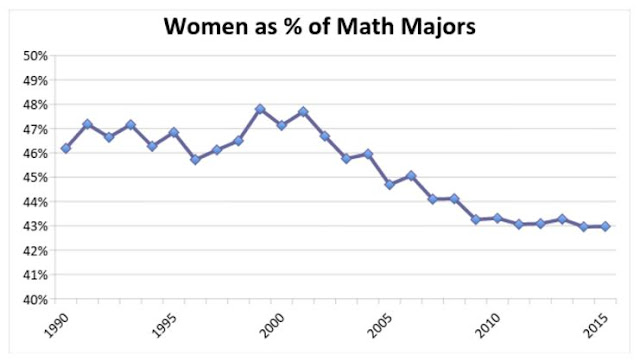Figure 2.1. Women as a percentage of all bachelor's degrees in the mathematical sciences. Note: Scale starts at 40%.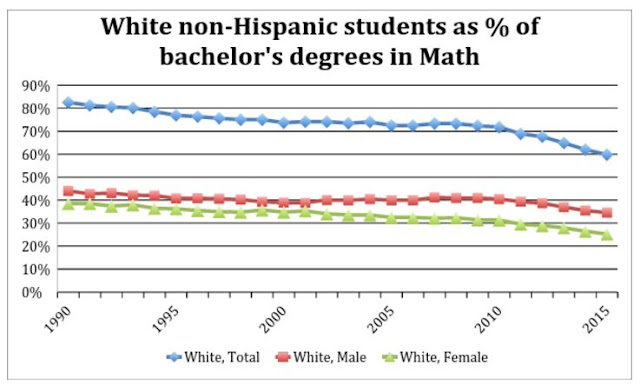Figure 2.2. White non-Hispanic students as a percentage of all bachelor's degrees in the mathematical sciences.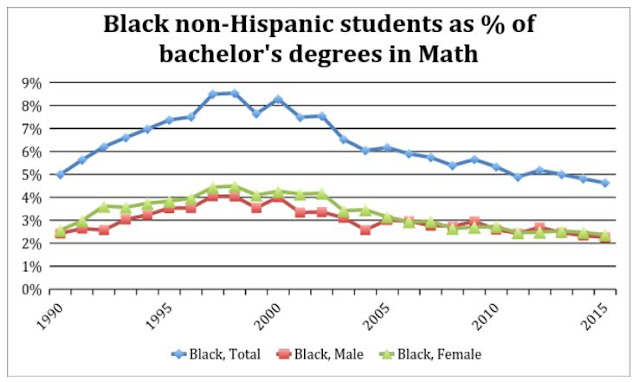Figure 2.3. Black non-Hispanic students as a percentage of all bachelor's degrees in the mathematical sciences.Figure 2.4. Hispanic students as a percentage of all bachelor's degrees in the mathematical sciences.Figure 2.5. Asian students as a percentage of all bachelor's degrees in the mathematical sciences.Figure 2.6. Non-Resident Alien students as a percentage of all bachelor's degrees in the mathematical sciences.

Appendix III. Bachelor’s degrees in the Physical Sciences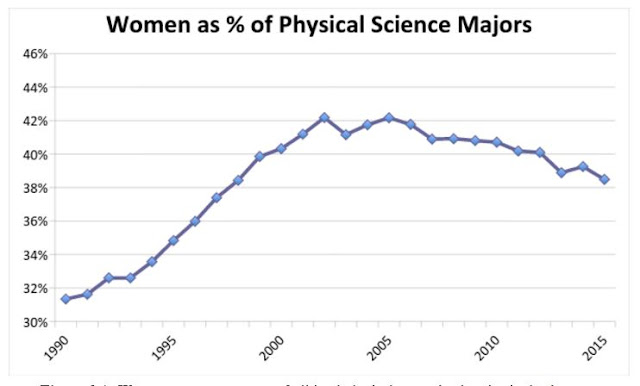Figure 3.1. Women as a percentage of all bachelor's degrees in the physical sciences. Note: scale starts at 30%Figure 3.2. White non-Hispanic students as a percentage of all bachelor's degrees in the physical sciences.Figure 3.3. Black non-Hispanic students as a percentage of all bachelor's degrees in the physical sciences.Figure 3.4. Hispanic students as a percentage of all bachelor's degrees in the physical sciences.Figure 3.5. Asian students as a percentage of all Bachelor's degrees in the physical sciences.Figure 3.6. Non-Resident Alien students as a percentage of all bachelor's degrees in the physical sciences.